Important: Please read the installation page for details about how to install the toolboxes. $\newcommand{\dotp}{\langle #1, #2 \rangle}$ $\newcommand{\enscond}{\lbrace #1, #2 \rbrace}$ $\newcommand{\pd}{ \frac{ \partial #1}{\partial #2} }$ $\newcommand{\umin}{\underset{#1}{\min}\;}$ $\newcommand{\umax}{\underset{#1}{\max}\;}$ $\newcommand{\umin}{\underset{#1}{\min}\;}$ $\newcommand{\uargmin}{\underset{#1}{argmin}\;}$ $\newcommand{\norm}{\|#1\|}$ $\newcommand{\abs}{\left|#1\right|}$ $\newcommand{\choice}{ \left\{ \begin{array}{l} #1 \end{array} \right. }$ $\newcommand{\pa}{\left(#1\right)}$ $\newcommand{\diag}{{diag}\left( #1 \right)}$ $\newcommand{\qandq}{\quad\text{and}\quad}$ $\newcommand{\qwhereq}{\quad\text{where}\quad}$ $\newcommand{\qifq}{ \quad \text{if} \quad }$ $\newcommand{\qarrq}{ \quad \Longrightarrow \quad }$ $\newcommand{\ZZ}{\mathbb{Z}}$ $\newcommand{\CC}{\mathbb{C}}$ $\newcommand{\RR}{\mathbb{R}}$ $\newcommand{\EE}{\mathbb{E}}$ $\newcommand{\Zz}{\mathcal{Z}}$ $\newcommand{\Ww}{\mathcal{W}}$ $\newcommand{\Vv}{\mathcal{V}}$ $\newcommand{\Nn}{\mathcal{N}}$ $\newcommand{\NN}{\mathcal{N}}$ $\newcommand{\Hh}{\mathcal{H}}$ $\newcommand{\Bb}{\mathcal{B}}$ $\newcommand{\Ee}{\mathcal{E}}$ $\newcommand{\Cc}{\mathcal{C}}$ $\newcommand{\Gg}{\mathcal{G}}$ $\newcommand{\Ss}{\mathcal{S}}$ $\newcommand{\Pp}{\mathcal{P}}$ $\newcommand{\Ff}{\mathcal{F}}$ $\newcommand{\Xx}{\mathcal{X}}$ $\newcommand{\Mm}{\mathcal{M}}$ $\newcommand{\Ii}{\mathcal{I}}$ $\newcommand{\Dd}{\mathcal{D}}$ $\newcommand{\Ll}{\mathcal{L}}$ $\newcommand{\Tt}{\mathcal{T}}$ $\newcommand{\si}{\sigma}$ $\newcommand{\al}{\alpha}$ $\newcommand{\la}{\lambda}$ $\newcommand{\ga}{\gamma}$ $\newcommand{\Ga}{\Gamma}$ $\newcommand{\La}{\Lambda}$ $\newcommand{\si}{\sigma}$ $\newcommand{\Si}{\Sigma}$ $\newcommand{\be}{\beta}$ $\newcommand{\de}{\delta}$ $\newcommand{\De}{\Delta}$ $\newcommand{\phi}{\varphi}$ $\newcommand{\th}{\theta}$ $\newcommand{\om}{\omega}$ $\newcommand{\Om}{\Omega}$

This tour explores the use of gradient descent method for unconstrained and constrained optimization of a smooth function

In :
addpath('toolbox_signal')


## Gradient Descent for Unconstrained Problems¶

We consider the problem of finding a minimum of a function $f$, hence solving $$\umin{x \in \RR^d} f(x)$$ where $f : \RR^d \rightarrow \RR$ is a smooth function.

Note that the minimum is not necessarily unique. In the general case, $f$ might exhibit local minima, in which case the proposed algorithms is not expected to find a global minimizer of the problem. In this tour, we restrict our attention to convex function, so that the methods will converge to a global minimizer.

The simplest method is the gradient descent, that computes $$x^{(k+1)} = x^{(k)} - \tau_k \nabla f(x^{(k)}),$$ where $\tau_k>0$ is a step size, and $\nabla f(x) \in \RR^d$ is the gradient of $f$ at the point $x$, and $x^{(0)} \in \RR^d$ is any initial point.

In the convex case, if $f$ is of class $C^2$, in order to ensure convergence, the step size should satisfy $$0 < \tau_k < \frac{2}{ \sup_x \norm{Hf(x)} }$$ where $Hf(x) \in \RR^{d \times d}$ is the Hessian of $f$ at $x$ and $\norm{\cdot}$ is the spectral operator norm (largest eigenvalue).

We consider a simple problem, corresponding to the minimization of a 2-D quadratic form $$f(x) = \frac{1}{2} \pa{ x_1^2 + \eta x_2^2, }$$ where $\eta>0$ controls the anisotropy, and hence the difficulty, of the problem.

Anisotropy parameter $\eta$.

In :
eta = 10;


Function $f$.

In :
f = @(x)( x(1)^2 + eta*x(2)^2 ) /2;


Background image of the function.

In :
t = linspace(-.7,.7,101);
[u,v] = meshgrid(t,t);
F = ( u.^2 + eta*v.^2 )/2 ;


Display the function as a 2-D image.

In :
clf; hold on;
imagesc(t,t,F); colormap jet(256);
contour(t,t,F, 20, 'k');
axis off; axis equal;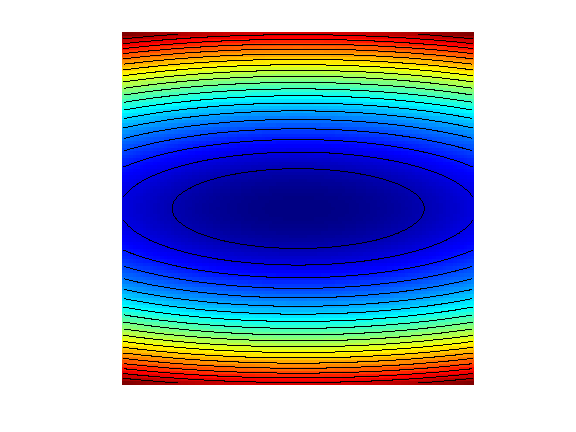In :
Gradf = @(x)[x(1); eta*x(2)];


The step size should satisfy $\tau_k < 2/\eta$. We use here a constrant step size.

In :
tau = 1.8/eta;


Exercise 1

Perform the gradient descent using a fixed step size $\tau_k=\tau$. Display the decay of the energy $f(x^{(k)})$ through the iteration. Save the iterates so that |X(:,k)| corresponds to $x^{(k)}$.

In :
exo1()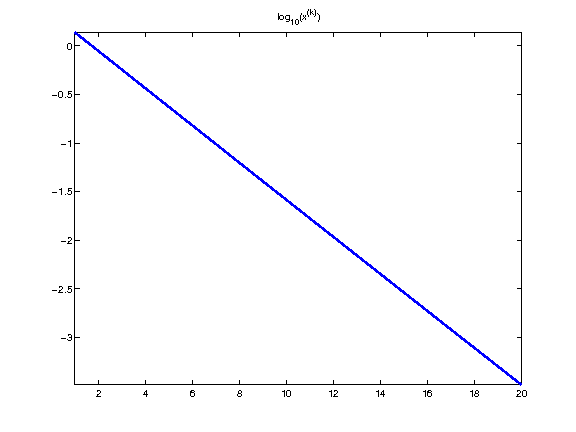In :
%% Insert your code here.


Display the iterations.

In :
clf; hold on;
imagesc(t,t,F); colormap jet(256);
contour(t,t,F, 20, 'k');
h = plot(X(1,:), X(2,:), 'k.-');
set(h, 'LineWidth', 2);
set(h, 'MarkerSize', 15);
axis off; axis equal;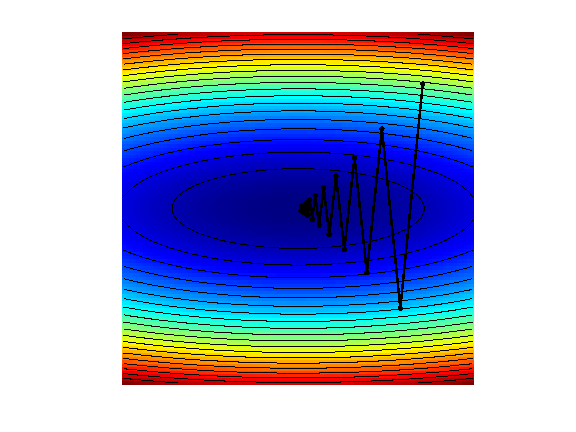Exercise 2

Display the iteration for several different step sizes.

In :
exo2()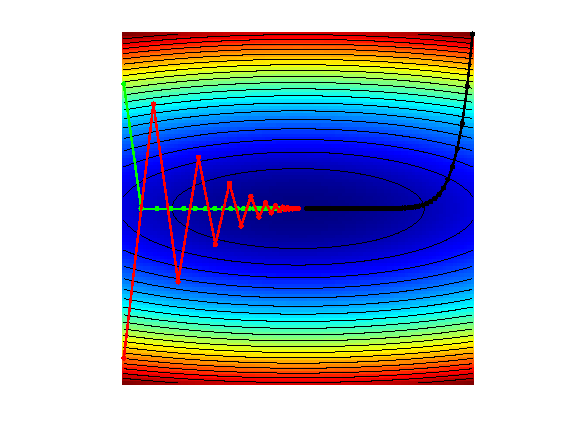In :
%% Insert your code here.


## Gradient and Divergence of Images¶

Local differential operators like gradient, divergence and laplacian are the building blocks for variational image processing.

Load an image $x_0 \in \RR^N$ of $N=n \times n$ pixels.

In :
n = 256;


Display it.

In :
clf;
imageplot(x0);For a continuous function $g$, the gradient reads $$\nabla g(s) = \pa{ \pd{g(s)}{s_1}, \pd{g(s)}{s_2} } \in \RR^2.$$ (note that here, the variable $s$ denotes the 2-D spacial position).

We discretize this differential operator on a discrete image $x \in \RR^N$ using first order finite differences. $$(\nabla x)_i = ( x_{i_1,i_2}-x_{i_1-1,i_2}, x_{i_1,i_2}-x_{i_1,i_2-1} ) \in \RR^2.$$ Note that for simplity we use periodic boundary conditions.

Compute its gradient, using finite differences.

In :
grad = @(x)cat(3, x-x([end 1:end-1],:), x-x(:,[end 1:end-1]));


One thus has $\nabla : \RR^N \mapsto \RR^{N \times 2}.$

In :
v = grad(x0);


One can display each of its components.

In :
clf;
imageplot(v(:,:,1), 'd/dx', 1,2,1);
imageplot(v(:,:,2), 'd/dy', 1,2,2);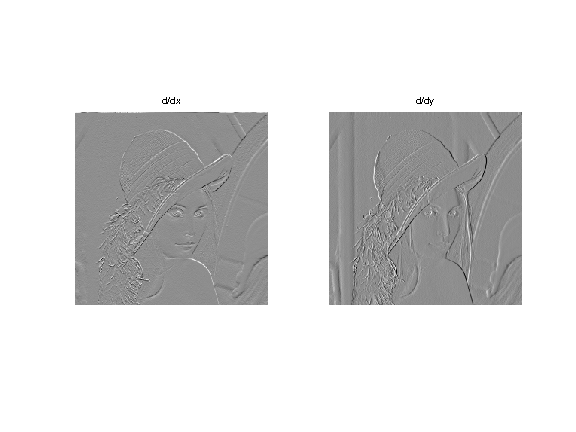One can also display it using a color image.

In :
clf;
imageplot(v);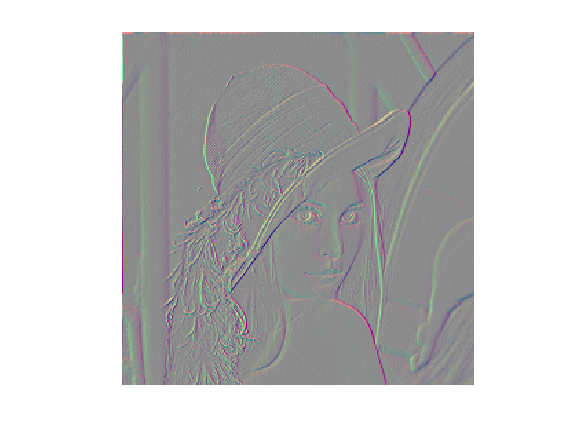One can display its magnitude $\norm{(\nabla x)_i}$, which is large near edges.

In :
clf;
imageplot( sqrt( sum3(v.^2,3) ) );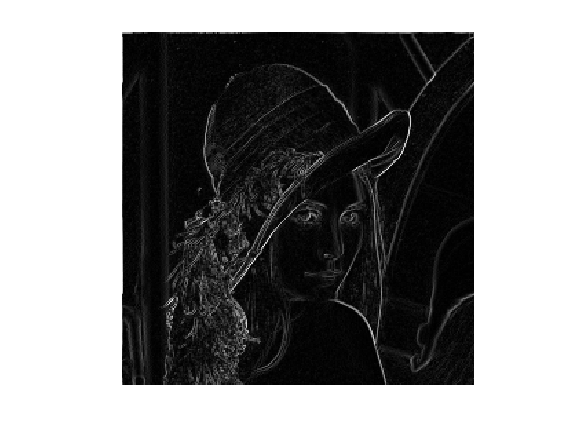The divergence operator maps vector field to images. For continuous vector fields $v(s) \in \RR^2$, it is defined as $$\text{div}(v)(s) = \pd{v_1(s)}{s_1} + \pd{v_2(s)}{s_2} \in \RR.$$ (note that here, the variable $s$ denotes the 2-D spacial position). It is minus the adjoint of the gadient, i.e. $\text{div} = - \nabla^*$.

It is discretized, for $v=(v^1,v^2)$ as $$\text{div}(v)_i = v^1_{i_1+1,i_2} - v^1_{i_1,i_2} + v^2_{i_1,i_2+1} - v^2_{i_1,i_2} .$$

In :
div = @(v)v([2:end 1],:,1)-v(:,:,1) + v(:,[2:end 1],2)-v(:,:,2);


The Laplacian operatore is defined as $\Delta=\text{div} \circ \nabla = -\nabla^* \circ \nabla$. It is thus a negative symmetric operator.

In :
delta = @(x)div(grad(x));


Display $\Delta x_0$.

In :
clf;
imageplot(delta(x0));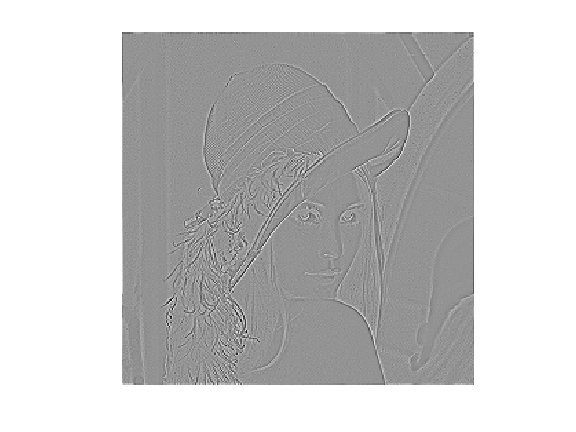Check that the relation $\norm{\nabla x} = - \dotp{\Delta x}{x}.$

In :
dotp = @(a,b)sum(a(:).*b(:));

Should be 0: 000


## Gradient Descent in Image Processing¶

We consider now the problem of denoising an image $y \in \RR^d$ where $d = n \times n$ is the number of pixels ($n$ being the number of rows/columns in the image).

Add noise to the original image, to simulate a noisy image.

In :
sigma = .1;
y = x0 + randn(n)*sigma;


Display the noisy image $y$.

In :
clf;
imageplot(clamp(y));